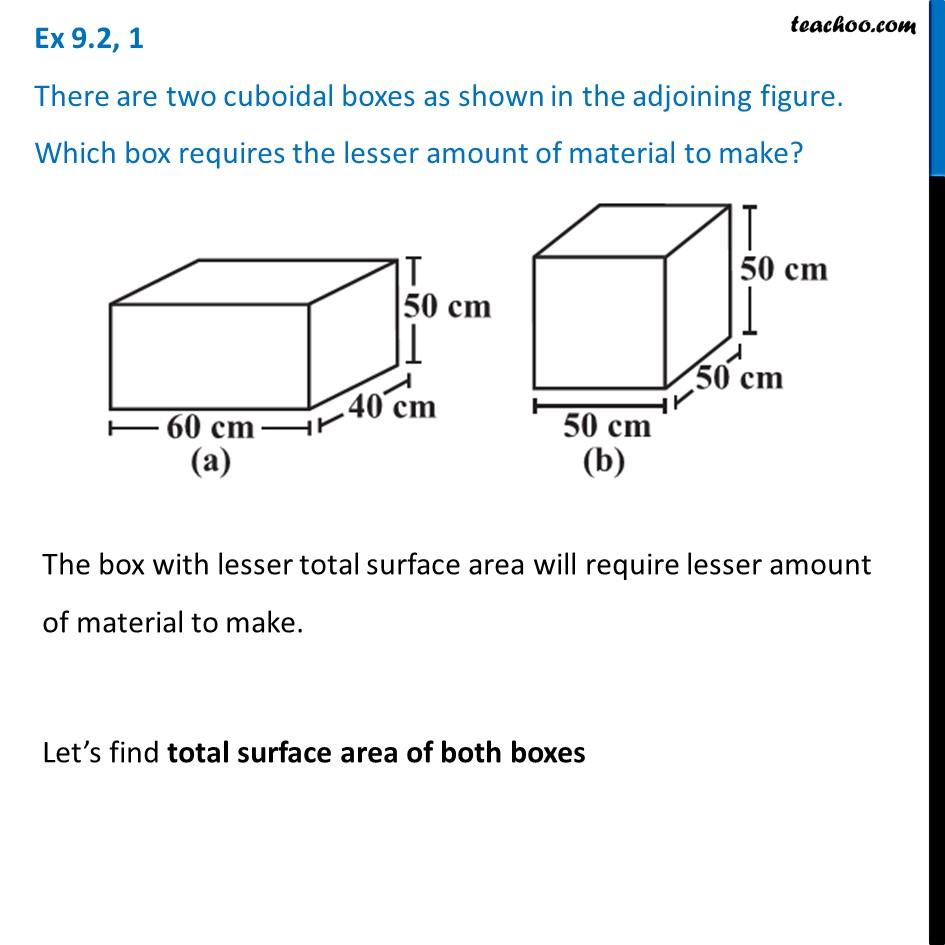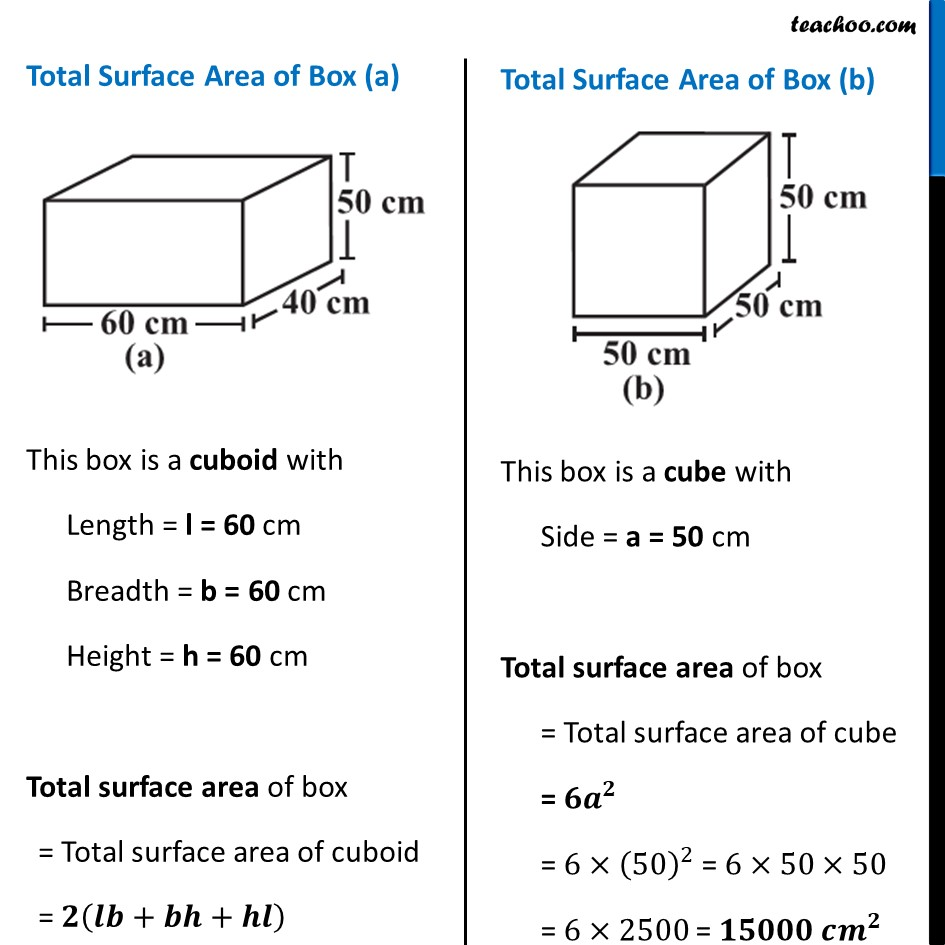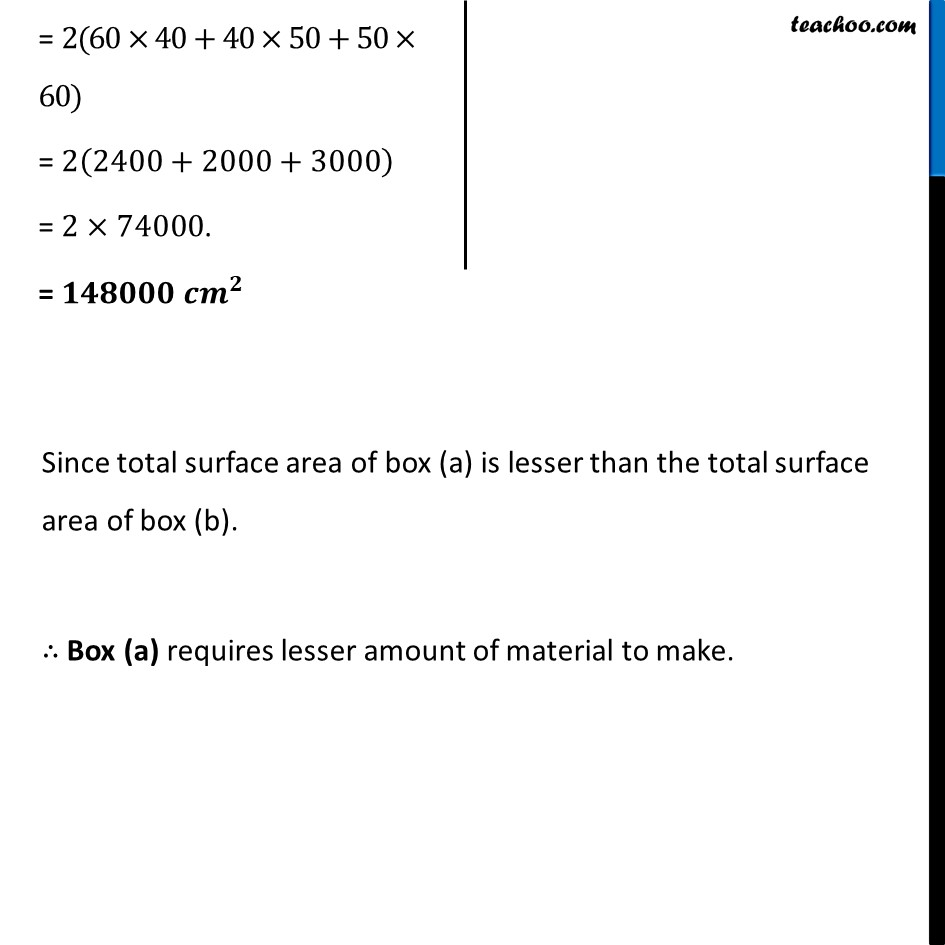Ex 9.2

Chapter 9 Class 8 Mensuration
Serial order wiseLearn in your speed, with individual attention - Teachoo Maths 1-on-1 Class

### Transcript

Ex 9.2, 1 There are two cuboidal boxes as shown in the adjoining figure. Which box requires the lesser amount of material to make?The box with lesser total surface area will require lesser amount of material to make. Let’s find total surface area of both boxes Total Surface Area of Box (a) This box is a cuboid with Length = l = 60 cm Breadth = b = 60 cm Height = h = 60 cm Total surface area of box = Total surface area of cuboid = 𝟐(𝒍𝒃+𝒃𝒉+𝒉𝒍) Total Surface Area of Box (b) This box is a cube with Side = a = 50 cm Total surface area of box = Total surface area of cube = 𝟔𝒂^𝟐 = 6×(50)^2 = 6×50×50 = 6×2500 = 𝟏𝟓𝟎𝟎𝟎 𝒄𝒎^𝟐 = 2(60×40+40×50+50×60) = 2(2400+2000+3000) = 2×74000. = 𝟏𝟒𝟖𝟎𝟎𝟎 𝒄𝒎^𝟐 Since total surface area of box (a) is lesser than the total surface area of box (b). ∴ Box (a) requires lesser amount of material to make.